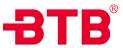• 系列号
F=灌装阀
• 功能
7=内封式
8=外封式
• 阀体材质
1=316L
2=316
3=304
• 执行器材质
5=304不锈钢
• 主要密封材质
9=PTFE(-20℃~+180℃)
1=PTFE复合(-20℃~+220℃)
• 执行器尺寸
03=32mm
• 接口
G=英制直管螺纹BSP DIN ISO228-1(国内G)
T=英制锥管螺纹BSPT DIN2999-1(国内Rc)
N=美制锥管螺纹NPTASMEB1.20.1
• 规格
-10=3/8"（DN10）
-15=1/2"（DN15）
-20=3/4"（DN20）
• 附件
-F3=2位3通电磁阀
F4=2位5通电磁阀